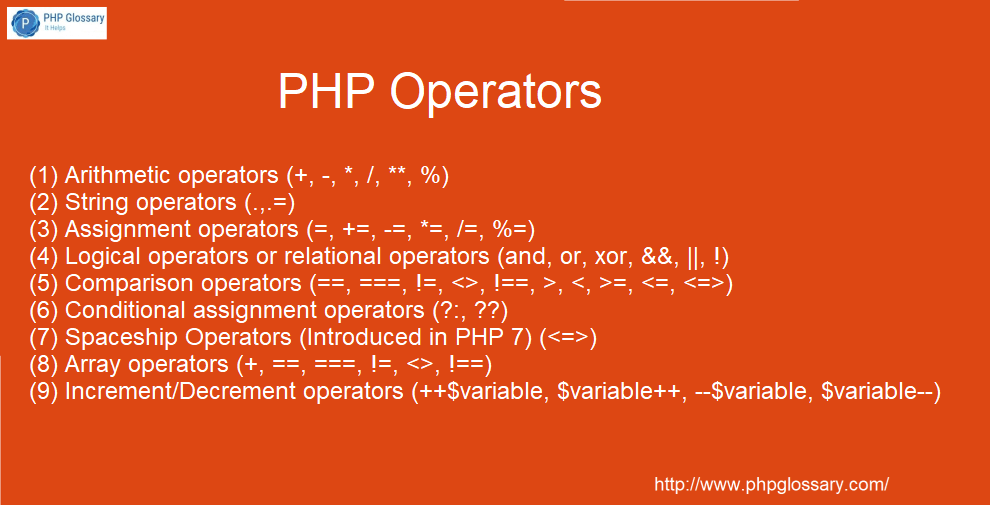PHP Tutorial

# PHP Operators and their usage with example code# PHP Operators

PHP operators are used to working on PHP variables & values and helps to get desire output.

PHP Operators are mainly a specific type of symbol that instructs the PHP to perform a particular operation.
e.g The add (+) symbol is an operator that informs PHP to add two or more variables or values, while the minus (-) symbol is an operator that informs PHP to subtract two or more values.

PHP has put the operators in the following categories:

1. Arithmetic operators (+, -, *, /, **, %)
2. String operators (.,.=)
3. Assignment operators (=, +=, -=, *=, /=, %=)
4. Logical operators or relational operators (and, or, xor, &&, ||, !)
5. Comparison operators (==, ===, !=, <>, !==, >, <, >=, <=, <=>)
6. Conditional assignment operators (?:, ??)
7. Spaceship Operators (Introduced in PHP 7) (<=>)
8. Array operators (+, ==, ===, !=, <>, !==)
9. Increment/Decrement operators (++\$variable, \$variable++, –\$variable, \$variable–)

## (1) Arithmetic operators (+, -, *, /, **, %)

To understand the use of arithmetic operators try the below example. Copy and paste the below-given PHP code in your PHP file and put it in your PHP Server’s document root and open it on the browser.

```<?php //(1)Arithmetic Operators (+, -, *, /, **, %)

\$number1 = 10;
\$number2 = 20;

// Plus(+) Operator
echo "Output of plus(+) operator : ";
echo \$number1+\$number2; // output : 30
echo "<br>"; // for new line or line break

// Substraction(Minus) operator
echo "Output of Minus(-) operator : ";
echo \$number2-\$number1; // output : 10
echo "<br>"; // for new line or line break

// Multiplication(*) operator
echo "Output of Multiplication(*) operator : ";
echo \$number1*\$number2; // output : 200
echo "<br>"; // for new line or line break

// Division(/) Operator
echo "Output of Division(/) operator : ";
echo \$number2/\$number1; // output : 2
echo "<br>"; // for new line or line break

// Exponentiation(**) operator
\$number3 = 3;
\$number4 = 4;

echo "Output of Exponentiation(**) operator : ";
echo \$number3**\$number4; // output : 81
echo "<br>"; // for new line or line break

// Modulus(%) operator
echo "Output of Modulus(%) operator : ";
echo \$number4%\$number3; // output : 1
echo "<br>"; // for new line or line break
?>```

The output of the above code: